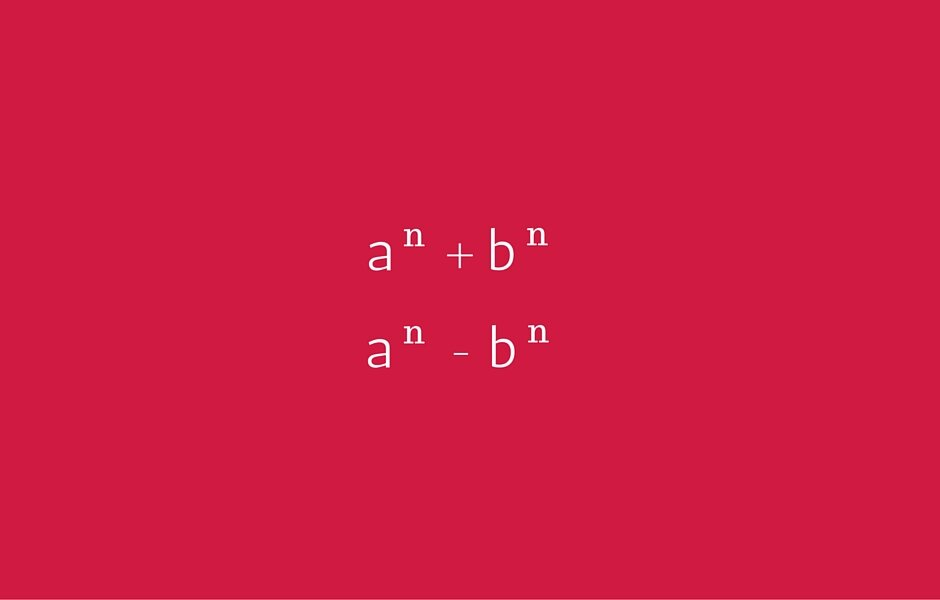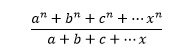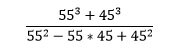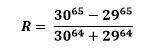Select PageOver the last few years, almost every CAT paper has had anywhere ranging from 1-2 questions from the topic involving the concept of special division type of either of an+bn or an-bn. While the concept is pretty easy to grasp and apply, many students make careless mistakes and assume a few things they shouldn’t both during prep and during the test.

General nature of the two expressions

The questions are primarily numerical and you have to find either the resultant value or the factor/s that divide the expression. It is not very difficult to spot these questions and they are not very time consuming either as you will see. If you are spending a lot of time calculating in these questions, as would be true with most of the other cases, you are doing it the wrong way. There are two basic things that you need to know to solve these questions and then it is just a matter of spotting the inlet and cracking the question.

When n is odd

• an+bn will be divisible by (a+b)
• an-bn will be divisible by (a-b)

When n is even

• an+bn does not show a particular trend
• an-bn will be divisible by (a+b)
• an-bn will be divisible by (a-b)

If a, b, c, d… are in an arithmetic progression and they are raised to an odd number, then the following expressionwill give a remainder of 0. In other words, (an+bn+cn…) is completely divisible by (a+b+c…) if n is an odd, positive integer.

Also, you have to understand the basic rules of addition and multiplication as they would be important while solving the questions in a quicker manner.

 First number Second number Product Sum Even Even Even Even Even Odd Even Odd Odd Odd Odd Even

You will see in a couple of examples below that once you understand the above mentioned basic concepts, you can solve these type of questions with minimal effort and a bit of visualization.

Examples from previous year CAT papers:

56 – 1 is divisible by? (CAT 1995)

(a) 13           (b) 31           (c) 5             (d) None of these

It can very well be seen that 56 – 1 can be written as 56 – 16

Probably the easiest way to do this is to expand the expression and solve it through options. But, a lot of students go for ‘shortcuts’ and end up marking the wrong answer.

As we have already seen, an – bn is always divisible by both (a+b) and (a-b) when n is even. So, we know that the expression is divisible by both 4 and 6 and so, none of these could be perceived to be the correct option. But then that is a trap that has been set to allure the careless aspirant into committing the fatal mistake.

If you look carefully, it can also be written as (253 – 13) and (1252 – 12) which would be divisible by 24 in the first case and by 124 and 126 in the second case. Now, as being divisible by 124 is the same as being divisible by 31 and 4 both, the answer would be option (b)

As you will see throughout the article series and also during my lectures, the idea of preparing for CAT is not to solve the toughest questions using shortcuts but to understand the minor traps that could be embedded into a paper making it look deceptively easy.

The value of the expression is (CAT 1995)(a) 100         (b) 105         (c) 125         (d) 75

This is a simpler question wherein, you have to expand (a3+b3) and so, you can simply mark the answer to be the quotient of (a3+b3) divided by (a2 – ab + b2) which would be (a+b) ie. 100

Let N = 553 + 173 – 723. N is divisible by (CAT 2000)

(a) both 7 and 13   (b) both 3 and 13   (c) both 17 and 7    (d) both 3 and 17

Again, you can either solve it completely or use options and divide the individual terms of the expression while getting remainders. Another way to do it is by using the above concepts.

We can rewrite the expression as 553-723+173

Considering 553-723 we understand that it would be divisible by 17. The other part is also divisible by 17 and so, the entire expression is definitely divisible by 17.

Similarly, if you consider 553+173, it will be divisible by 72. The other part is also divisible by 72 and so, the entire expression is divisible by 72 and so, by 3.

So, option (d) would be correct. You can see that once you understand the concept, application is extremely straightforward and this question could have been solved orally.

A few other questions that appeared in previous year CAT papers.

Let b be a positive integer and a = b2 – b. If b ≥ 4, then a2 – 2a is divisible by

(a) 15           (b) 20           (c) 24           (d) None of these

The obvious option is to go for trial and error and find out the answer. But if someone is technically bent and wants to understand the science behind it, you can follow this train of thought.

a=b(b-1)

So, a2-2a will be equal to

a(a-2)

b(b-1)(b2-b-2)

b(b-1)(b-2)(b+1)

This is simply the product of 4 consecutive integers. Four consecutive integers will always have two consecutive even and two consecutive odd integers and so, at least one multiple of 2, 3 and 4 each. So, the end product will be divisible by 24.

76n – 66n, where n is an integer > 0, is divisible by (CAT 2003)

(a) 13           (b) 127         (c) 559         (d) All of these

(73n + 63n)(73n – 63n)

(343n + 216n)(343n – 216n)

If n is odd, the expression will be divisible by 559 and 127

If n is even, the expression will be divisible by 559 and 127

Also, if you observe carefully, 559 = 13*43

So, option (d) is correct.

A few questions that you can practice:

The remainder, when (1523 + 2323) is divided by 19, is (CAT 2004)

(a) 4            (b) 15          (c) 0            (d) 18

If x = (163 + 173 + 183 + 193), then x divided by 70 leaves a remainder of (CAT 2005)

(a) 0            (b) 1            (c) 69          (d) 35

What is the value of R in the given expression (CAT 2005)(a) 0 < R ≤ 0.1       (b) 0.1 < R ≤ 0.5    (c) 0.5 < R ≤ 1.0    (d) R > 1.0

Final words

The special division questions looks deceptively simple and one might simply leave a question during practice assuming that ‘substitution’ would work during the test and the question is elementary. But, on close viewing, if you are not really sure of the question type, it can take you a lot longer to solve these type of questions and so, an extra moment spent in actually solving the question during prep would be extremely valuable.

All the best!

error: Content is protected !!